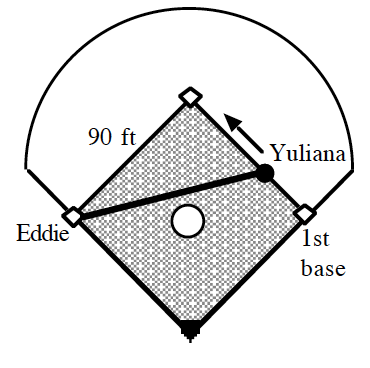### Home > CALC > Chapter 7 > Lesson 7.2.4 > Problem7-100

7-100.

Eddie is standing on third base, watching in amazement as Yuliana tries to steal second. If Yuliana runs at $15\frac {\text{ ft }} { \operatorname { cos } }$, how fast is her distance from Eddie changing when she is $40$ feet from first base?

Notice the right triangle.
Let $x =$ Yuliana's distance from ____________ base.
Find the remaining two sides of the triangle, in terms of $x$.

Things that change (or describe change) should be 'plugged in' to the derivative equation, not the original equation . This includes Yuliana's running rate and her position from 1st base at the moment in question.

Is Yuliana's rate positive or negative?

The distance between Yuliana and Eddie is changing at a rate of $-7.285$ ft/sec when Yulanda was $40$ feet from 1st base.